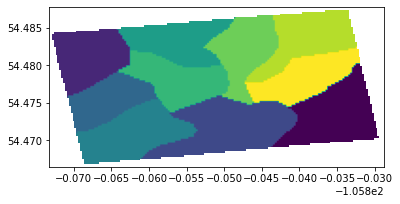# bounding_box#

You can find instructions to open a geoTIFF file here. Instructions on how to download and install Rasterio and GDAL(not necessary for linux) are included too.

In addition to that, we also need to install matplotlib and Shapely in order to clip the raster.

To install matplotlib and Shapely, run the commands :

```pip install matplotlib
```
```pip install shapely
```

Import the required libraries

```import rasterio as rst
from rasterio.plot import show
import matplotlib.pyplot as plt
import numpy as np
```

Store the geoTIFF file in a variable, use the rs.open() and show() functions to open view the image in the next cell.

We can also perform different operations using raster objects: the number of bands, the image resolution, CRS value, no-data value, number of raster bands, etc.

```bounding_box = r'bounding_box.tiff'
img = rst.open(bounding_box)
show(img)
#No. of bands
print(img.count)

#Image resolution
print(img.height, img.width)

# CRS
print(img.crs)

# No-data
print(img.nodata)
``````1
85 178
EPSG:4326
255.0
```